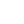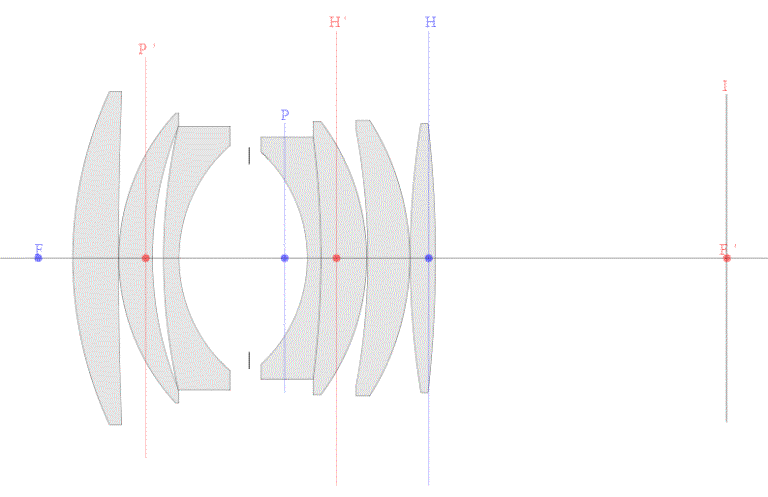----------- Your trusted source for independent sensor data- Photons to Photos------------ Last revised: 2018-02-26 15:00 GMT-5

Previous Article----------------------------------- Table of Contents------------------------------------ Next Article

# ------------------- Optics Primer - F-number and Numerical Aperture

--------------------------------------------------------- By Bill Claff

Many readers are aware that the diameter of the entrance pupil relates to the f-number (f#) of the lens. The formula is:
PD = f / f# or f# = f / PD
where PD is the entrance pupil diameter, f is the focal length, and f# is the f-number.
This is an object space, light gathering property.

Mathematically the entrance and exit pupils are conjugates. In practical terms this simply means that all the light gathered by the entrance pupil is delivered to the exit pupil.

In image space the half-angle the exit pupil makes with the image plane determines Numerical Aperture (NA). The formula is:
NA = sin q
where NA is Numerical Aperture and q is the half-angle.

Numerical Aperture and f-number are related by this equation:
f# = 1 / (2 * NA) or NA = 1 / (2 * f#)

Strictly speaking the is the real definition of f-number whereas f / PD is a close approximation.
So, effective f-number and Numerical Aperture are closely related.

In any case, we can use the entrance pupil diameter from our ray tracing to calculate f-number at infinity and the exit pupil diameter at other than infinity to calculate NA and effective f-number.
(Because the lens specifications don't state an aperture diameter I actually tune the aperture diameter to meet the specification at infinity.)

Pupil magnification is the ratio of exit pupil diameter to entrance pupil diameter:
p = P'D / PD
Note that pupil magnification can be less than one. Values tend to be less than 1 at longer focal lengths and greater than 1 at short focal lengths.
Because of geometry (similar triangles) effective f-number is normally stated in terms of pupil magnification rather than Numerical Aperture.
Pupil magnification and effective f-number are normally only important in close-up photography.

Here's another look at the 50mm lens with cardinal points and the pupils:For this lens the pupil magnification is close to 1.5
Note that P' is 1.5 times are far away from F' as H' and note that P is 1/1.5 times as far away from F as H.

Finally, for now, the entrance pupil is the center of perspective.
To accomplish multi-image panoramic photographic, particularly at short distances, you must rotate the lens around the entrance pupil to maintain perspective.
(Ignoring the nuance that entrance pupil, especially for wide angle lenses, moves with viewing angle!)